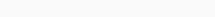# Math Puzzles Puzzle 13 Answer AndroidMath puzzle game level 13 solution with detailed hints and explanation, Scroll below to find out.

Math puzzles increase your logical thinking. The objective of the game is to find patterns hidden in the puzzle in order to arrive at the solution. Challenge yourself with different levels of math puzzles and stretch the limits of your intelligence.

### Math Puzzles Puzzle 13:

9-3/3*5+10=?Solution/Hint: Apply Bodmas

first, do division and multiplication

then sum and subtraction

If there is any Doubt or you are unable to understand the solution, then please let me know in comments, I will surely help you.

### 3 thoughts on “Math Puzzles Game Level 13 Answer with Solution”

•April 11, 2020 at 03:10

I believe you are wrong on this one
If you apply bodmas
Therefore answer will be negative 6
I believe its algebraic

•June 19, 2021 at 23:48
•